# Parallel Foreach Loop in C#

## Parallel Foreach Loop in C#

In this article, I am going to discuss the Parallel Foreach Loop in C# with Examples.  As we already discussed in our previous article that the Task Parallel Library (TPL) provides two methods (i.e. Parallel.For and Parallel.Foreach) which are conceptually the “for” and “for each” loops, except that, they use multiple threads to execute multiple iterations at the same time on a machine with multiple cores. In our previous article, we already discussed the Parallel for Method in C# with examples. Here, in this article, I am going to keep the focus on the Parallel Foreach method in C#.

##### Parallel.ForEach Loop in C#

Parallel Foreach is the equivalent of a normal foreach, which can occur in parallel. It is useful when we want to iterate through a collection and we need to do relatively hard work on each item in the collection. It should be clear that the work we need to do is CPU bound since we have already seen that it is the ideal type of work for the parallel class.

The Parallel ForEach in C# provides a parallel version of the standard, sequential Foreach loop. In a standard Foreach loop, each iteration processes a single item from the collection and will process all the items one by one only. However, the Parallel Foreach method executes multiple iterations at the same time on different processors or processor cores. This may open the possibility of synchronization problems. So, the loop is ideally suited to processes where each iteration is independent of the others.

Note: We need to use parallel loops such as Parallel.For and Parallel.ForEach method to speed up operations where an expensive, independent CPU-Bound operation needs to be performed for each input of a sequence.

##### A sequential Foreach Loop Syntax in C#: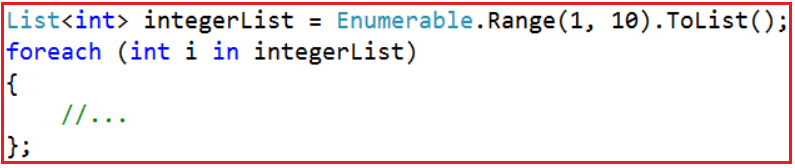##### A Parallel Foreach Loop Syntax in C#: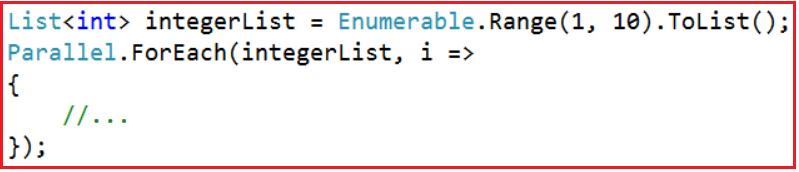The parallel version of the loop uses the static ForEach method of the Parallel class. There are many overloaded versions available for this method. This is the simplest overloaded version that accepts two arguments. The first one is the collection of objects that will be enumerated. This can be any collection that implements IEnumerable<T>.

The second parameter accepts an Action delegate, usually expressed as a lambda expression that determines the action to take for each item in the collection. The delegate’s parameter contains the item from the collection that is to be processed during the iteration.

##### Parallel Foreach Loop Example in C#

Let us understand Parallel Foreach Method with an example. First, we will write an example using the standard sequential Foreach loop and will see how much time it will take to complete the execution. Then we will write the same example using the Parallel ForEach Loop method and will see how much time it will take to complete the execution of the same example.

In the below example, we create a sequential Foreach Loop that performs a long-running task once for each item in the collection. The code below loops through a list of ten integers generated using the Enumerable.Range method. In each iteration, the DoSomeIndependentTimeconsumingTask method is called. The DoSomeIndependentTimeconsumingTask method performs a calculation that is included to generate a long enough pause to see the performance improvement of the parallel version.

##### Example using Standard Foreach Loop in C#:
```using System;
using System.Collections.Generic;
using System.Diagnostics;
using System.Linq;
namespace ParallelProgrammingDemo
{
class Program
{
static void Main()
{
Stopwatch stopwatch = new Stopwatch();

Console.WriteLine("Standard Foreach Loop Started");
stopwatch.Start();
List<int> integerList = Enumerable.Range(1, 10).ToList();
foreach (int i in integerList)
{
Console.WriteLine("{0} - {1}", i, total);
};

Console.WriteLine("Standard Foreach Loop Ended");
stopwatch.Stop();

Console.WriteLine(\$"Time Taken by Standard Foreach Loop in Miliseconds {stopwatch.ElapsedMilliseconds}");
}

{
//Do Some Time Consuming Task here
long total = 0;
for (int i = 1; i < 100000000; i++)
{
total += i;
}
}
}
}```

Now run the application and observe the output.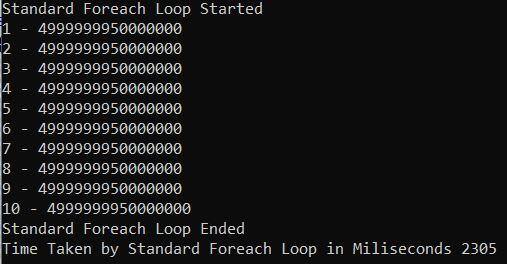As you can see from the above output the standard Foreach Loop statement took approximately 2305 milliseconds to complete the execution. Let’s rewrite the same example using the C# Parallel ForEach method.

##### Example using Parallel Foreach Loop in C#:

Let’s rewrite the previous example using the Parallel ForEach Loop and see the output.

```using System;
using System.Collections.Generic;
using System.Diagnostics;
using System.Linq;

namespace ParallelProgrammingDemo
{
class Program
{
static void Main()
{
Stopwatch stopwatch = new Stopwatch();

Console.WriteLine("Parallel Foreach Loop Started");
stopwatch.Start();
List<int> integerList = Enumerable.Range(1, 10).ToList();

Parallel.ForEach(integerList, i =>
{
Console.WriteLine("{0} - {1}", i, total);
});
Console.WriteLine("Parallel Foreach Loop Ended");
stopwatch.Stop();

Console.WriteLine(\$"Time Taken by Parallel Foreach Loop in Miliseconds {stopwatch.ElapsedMilliseconds}");
}

{
//Do Some Time Consuming Task here
long total = 0;
for (int i = 1; i < 100000000; i++)
{
total += i;
}
}
}
}```

Now, run the above code and see the output as shown below. The time may vary on your machine.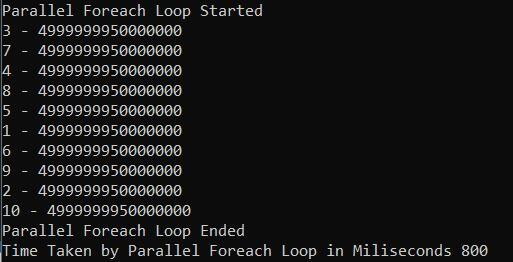As you can see in the above output, the Parallel.ForEach method took 800 milliseconds to complete the execution as compared with 2305 milliseconds with the standard Foreah loop in C#.

##### Using Degree of Parallelism in C# with Parallel Foreach Loop:

Using the Degree of Parallelism in C# we can specify the maximum number of threads to be used to execute the parallel foreach loop. The syntax to use the Degree of Parallelism in C# is given below.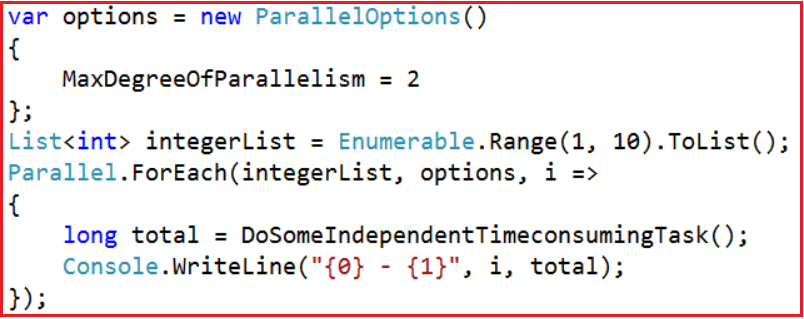The MaxDegreeOfParallelism property affects the number of concurrent operations run by Parallel method calls that are passed to this ParallelOptions instance. A positive property value limits the number of concurrent operations to the set value. If it is -1, there is no limit on the number of concurrently running operations.

By default, For and ForEach will utilize however many threads the underlying scheduler provides, so changing MaxDegreeOfParallelism from the default only limits how many concurrent tasks will be used.

##### Example to Understand Degree of Parallelism in C#

Let us see an example for a better understanding. In the below example, we are executing the Parallel Foreach method without using Degree of Parallelism. That means we are not limiting the number of threads to execute the Parallel Foreach method.

```using System;
using System.Collections.Generic;
using System.Linq;
namespace ParallelProgrammingDemo
{
class Program
{
static void Main()
{
List<int> integerList = Enumerable.Range(0, 10).ToList();
Parallel.ForEach(integerList, i =>
{
});
}
}
}```
###### Output: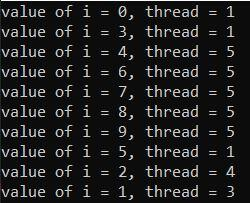Now run the above code multiple times, and definitely, you will get different output. You will also observe that the number of threads created is not in our control. In my case, 4 threads are used to run the parallel foreach loop. In your case, the number of threads might vary. Now, let us see how to restrict the number of threads to be created.

##### How to control the degree of concurrency i.e. How to restrict the number of threads to be created?

We can restrict the number of concurrent threads created during the execution of a parallel loop by using the MaxDegreeOfParallelism property of ParallelOptions class. By assigning some integer value to MaxDegreeOfParallelism, we can restrict the degree of this concurrency and can restrict the number of processor cores to be used by our loops. The default value of this property is -1, which means there is no restriction on concurrently running operations.

##### Example using Degree of Parallelism in C# to Restrict the number of Threads

In the below example, we have set MaxDegreeOfParallelism to 2 which means a maximum of 2 threads are going to execute our parallel foreach loop.

```using System;
using System.Collections.Generic;
using System.Linq;
namespace ParallelProgrammingDemo
{
class Program
{
static void Main()
{
List<int> integerList = Enumerable.Range(0, 10).ToList();
var options = new ParallelOptions() { MaxDegreeOfParallelism = 2 };

Parallel.ForEach(integerList, options, i =>
{
});
}
}
}```

Now run the application and see the output as shown below. Whatever number of times we execute the above code, the number of threads will never go above 2.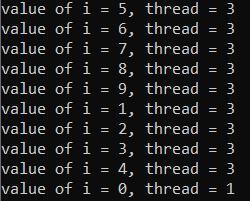##### Speed Benefits of Parallelism in C#:

We have already understood that increasing speed is the most important reason for using parallelism. We have seen several examples where we compare the sequential and parallel execution of an algorithm, and we have always seen a decrease in the execution time of the program by using parallelism. In other words, we have always obtained better results when using parallelism.

However, as we know, nothing is free in this life and parallelism is not the exception. We will not always obtain better results when introducing parallelism in our applications. This is because there is a cost to preparing the use of multithreading. That is why it is always advisable to take measurements to see whether the use of parallelism exceeds the cost.

##### Is it Worth using Parallelism in C#?

We can make an analogy. If you are a teacher who has to correct one exam and let’s say that it takes you four minutes to correct a test. Let’s also assume that finding two helpers takes 45 minutes and each helper takes four minutes to correct the exam.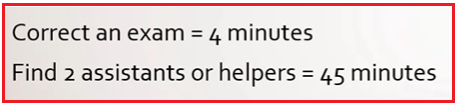Is it worth hiring a helper for this task? If you spend 45 minutes finding two helpers or two assistants and then give the task to one of them to correct that, it will take him 4 minutes to correct that, then the total time of the task adding the 45 minutes of looking for the assistance and four minutes of correcting this time adds up to 49 minutes, which is more than the four minutes it would have taken you to correct the exam by yourself.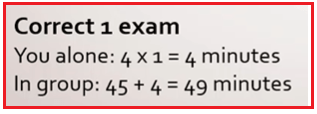As you can see, working with assistants took more time than working alone. The cost of this is the small number of tests to correct. Suppose that instead of one exam there were 150 exams. So on your own or alone it will take you 600 minutes to correct them. But together with your assistants, the time will be only 245 minutes.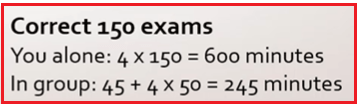As you can see in the second case, having the systems paid off, even taking into account the 45 minutes it took to hire those assistants.

Something similar happens with Parallelism. Sometimes the work to do is so small, so little that it is faster to use Sequential Programming and not Parallel Programming. The important thing is to take measurements before and after introducing parallelism to make sure that parallelism really pays off.

##### Example For a Better Understanding:

Please have a look at the below example. In the below example, the same task is going to be performed using both C# Standard For Loop and Parallel Foreach Loop. But here the task is not an expensive or time-consuming task. It is just a simple task. Now, if you run the code you will observe that the Parallel version of the foreach loop takes more time as compared to the standard foreach loop. This is because the parallel foreach creates multiple threads which will take some time which is not in the case of a standard foreach loop as a single thread is going to execute the tasks.

```using System;
using System.Collections.Generic;
using System.Diagnostics;
using System.Linq;

namespace ParallelProgrammingDemo
{
class Program
{
static void Main()
{
Stopwatch stopwatch = new Stopwatch();

Console.WriteLine("Standard Foreach Loop Started");
stopwatch.Start();
List<int> integerList = Enumerable.Range(1, 10).ToList();
foreach (int i in integerList)
{
};

stopwatch.Stop();
Console.WriteLine("Standard Foreach Loop Ended");
Console.WriteLine(\$"Time Taken by Standard Foreach Loop in Miliseconds {stopwatch.ElapsedMilliseconds}");

Console.WriteLine("\nParallel Foreach Loop Started");
stopwatch.Restart();

Parallel.ForEach(integerList, i =>
{
});

stopwatch.Stop();
Console.WriteLine("Parallel Foreach Loop Ended");

Console.WriteLine(\$"Time Taken by Parallel Foreach Loop in Miliseconds {stopwatch.ElapsedMilliseconds}");

}

{
Console.WriteLine(\$"Number: {i}");
}
}
}```
###### Output: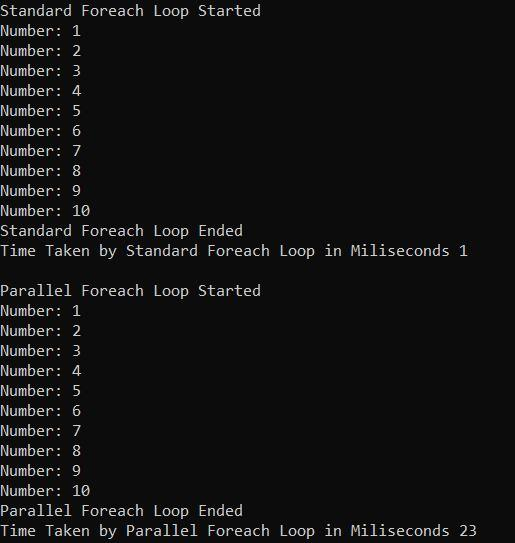As you can see in the above image, in my machine, the standard forerach loop took 1 second compared with 23 seconds with the Parallel foreach loop. So, this proves that the Parallel Foreach loop does not always give you better performance. So, you have to take measurements before and after introducing parallelism to make sure that parallelism really gives you better performance.

In our application, it is clear that we can do both. Some parts of our software may use parallelism and other parts may use sequential programming. The important thing is to have performance that justifies the decisions we make in our application.

In the next article, I am going to discuss the Parallel Invoke Method in C# with Examples. Here, in this article, I try to explain the Parallel ForEach in C# with Examples. I hope you understood the need and use of Parallel.Foreach Method in C#.

### 2 thoughts on “Parallel Foreach Loop in C#”

1.1.